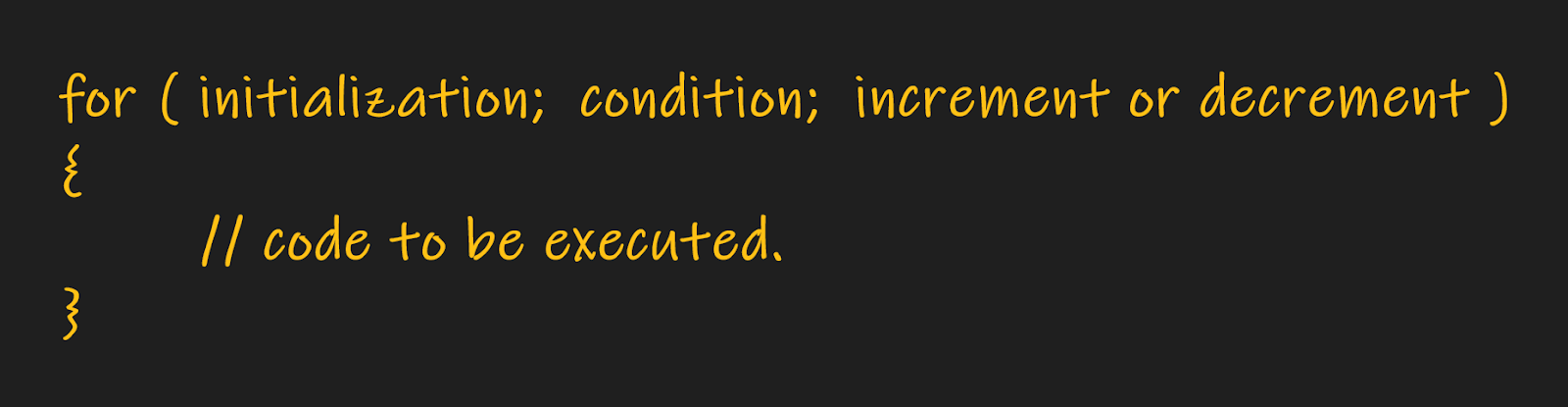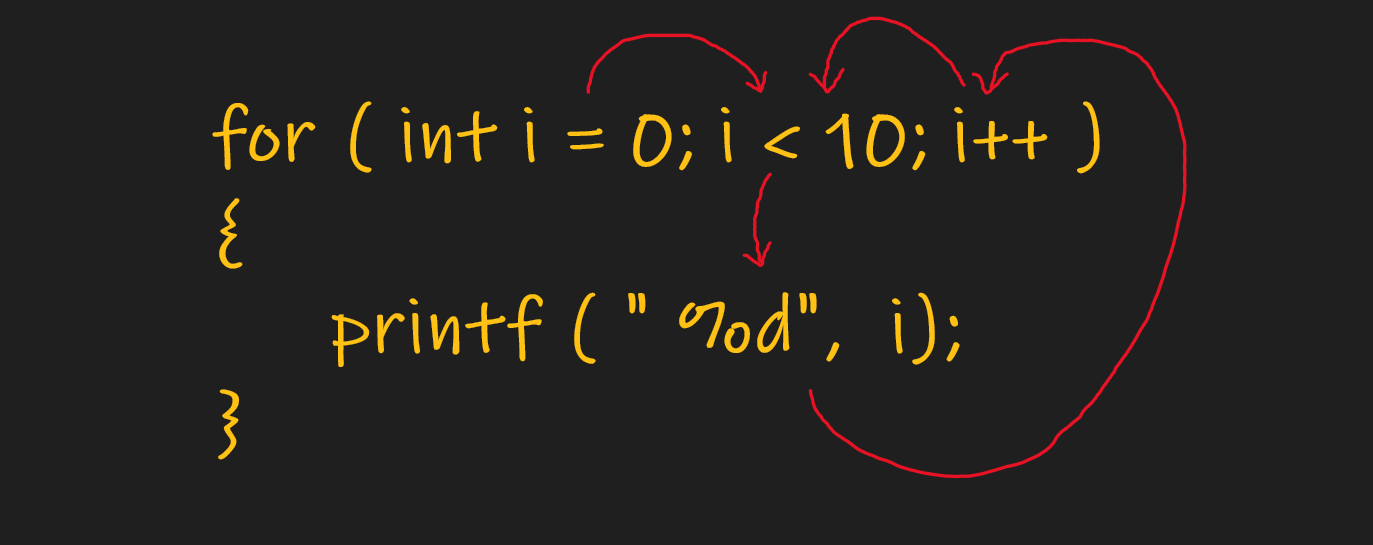# for loop in C++

Problem Statement: C++ for loop. Explanation, Syntax, Initialization, working, Etc.

In C++, and all other modern programming languages, The statements which are used to execute iteration are called loops, Loops allow a set of instructions to be repeatedly executed until a certain condition is reached.

In C++ the For loop condition is predetermined.

In another way we can say, A loop can be used to tell a program to execute statements repeatedly. Or we can say that a loop repeatedly executes the same set of instructions until a termination condition is met.

C++ For loop is helpful while writing complex logic and reducing the size of large programs.

The functional aspect of all the loops is the same, the only syntax is different.

### Syntax:-

Now let’s understand the Syntax of for loop in c++.

## Three things are very important in the loop

1. First is the initialization.
2. Second is Condition / Termination.
3. The third is Increment / Decrement.
``

Examples:

Example 1: Run a for loop in c++ to print the numbers between the range of 1 to 9.

```Input: None
Output: 1 2 3 4 5 6 7 8 9
Explanation:
Let’s Understand the flow of the Loop.```

The above code will print the numbers in the range of 1 to 9.

Let’s understand what is happening inside the loop.

1. First Computer reaches the part of initialization that is ‘i’ equal to 1.
2. Next, the computer checks the termination condition. I.e Computer checks whether the ‘i’ is less than 10 or not. but currently, the value of ‘i’ is 1, So 1 is definitely less than 10. The result is True.
3. Now, The computer executes the code which is present inside the block of the for a loop.
4. After completing the execution of the code which is inside the loop the control flow goes to the Increment / Decrement condition of the loop.
5. Now, It performs the Increment / Decrement operation (i.e. Now ‘i’ becomes 2 as we are incrementing the ‘i’ value by one i++).
6. After that, the computer again checks the termination condition (i.e If ‘i’ is less than 10 or not). Currently the condition is true i.e  2 < 10.
7. Now, The computer executes the code which is present inside the block of the for a loop. And this loop continues to run until the termination condition becomes false.
8. Once the condition becomes false i.e ‘i’ becomes greater than or equal to 10. The loop terminates and the computer starts the execution of the code which is present below the for a loop.

Code:

## C++ Code

``````#include <iostream>
using namespace std;
int main() {
for (int i = 0; i < 10; i++) {
cout << i << " ";
}
cout << endl;
}
``````

Output:- 1 2 3 4 5 6 7 8 9

Example 2:

Run a for loop in c++ to print all the even numbers between the given range (Left and Right). Given that both L and R even numbers.

```Input: L = 2, R = 10
Output: 2 4 6 8 10
Explanation: Everything is the same as explained in the above example, Just a minor change here is that the increment condition is incrementing by 2, Instead of 1.
```

Code:

## C++ Code

``````#include <iostream>
using namespace std;
int main() {
int l=2, r=10;

for (int i = l; i <= r; i += 2) {
cout << i << " ";
}
cout << endl;
}
``````

Output: 2 4 6 8 10

Conclusion: Many complex problems can be solved easily with the help of for loop in c++. So understanding the concept of loops is a must.